Question

# If the inflation rate is 3% and the nominal interest rate is 8%, what is the...

1. If the inflation rate is 3% and the nominal interest rate is 8%, what is the real interest rate? Your answer is referred to as the real pre‐tax interest rate.

Based on the Fischer relation,

(1 + Nominal Rate) = (1 + Real Rate) * (1 + Inflation)

(1 + 8%) = (1 + Real rate) * (1 + 3%)

(1 + Real Rate) = 1.08/1.03 = 1.0485

Real Rate = 4.85%

#### Earn Coins

Coins can be redeemed for fabulous gifts.

Similar Homework Help Questions
• ### The nominal interest rate is 8 percent and the inflation rate is 3 percent. What is...

The nominal interest rate is 8 percent and the inflation rate is 3 percent. What is the real interest rate? a. 8 percent b. 2 percent c. 11 percent d. 5 percent

• ### Suppose the real interest rate is 3% and expected inflation is 3%, what is the nominal...

Suppose the real interest rate is 3% and expected inflation is 3%. What is the nominal interest rate?nominal interest rate: = _______ %All else equal, if inflation decreases by 0 %, what will happen to the nominal interest rate?The real interest rate will decrease by 0 %.The nominal interest rate will decrease by 0 %.The nominal interest rate will increase by 0 %.The real interest rate will increase by 0 %.What do economists call the relationship between the nominal interest...

• ### Suppose the real interest rate is 3% and expected inflation is 3%. What is the nominal...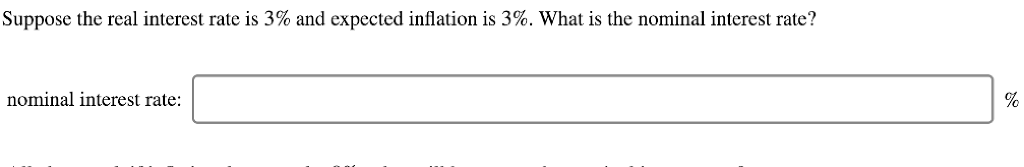Suppose the real interest rate is 3% and expected inflation is 3%. What is the nominal interest rate? nominal interest rate:

• ### If the nominal interest rate is 7% and the inflation rate is 2%, what is the...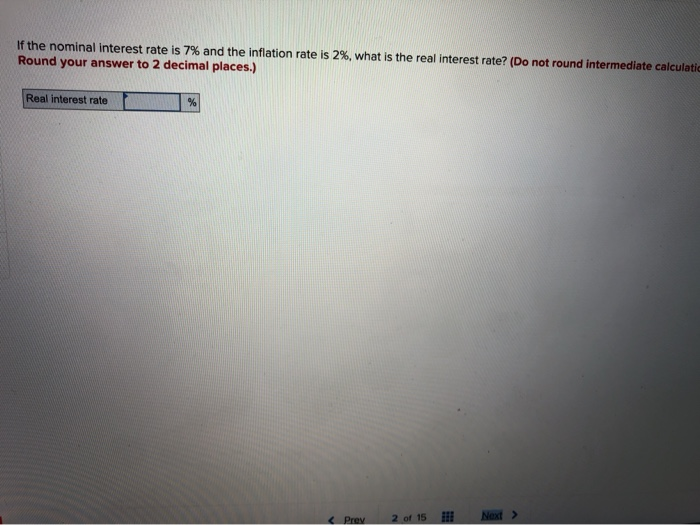If the nominal interest rate is 7% and the inflation rate is 2%, what is the real interest rate? (Do not round intermediate calculati Round your answer to 2 decimal places.) Real interest rate Next > Prey 2 of 15

• ### If the rate of inflation is 5%, what nominal interest rate is necessary for you to...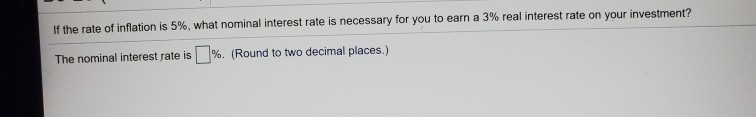If the rate of inflation is 5%, what nominal interest rate is necessary for you to earn a 3% real interest rate on your investment? The nominal interest rate is %. (Round to two decimal places.)

• ### The nominal interest rate is 12%. The inflation rate is 3%. The real interest rate is...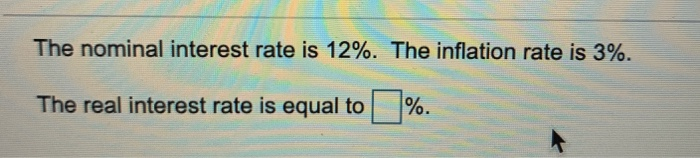The nominal interest rate is 12%. The inflation rate is 3%. The real interest rate is equal to %.

• ### If a nominal interest rate is 8 percent, and inflation is 8 percent, then the real...

If a nominal interest rate is 8 percent, and inflation is 8 percent, then the real interest rate is: minus 2 percent. 16 percent. 0 percent. 8 percent.

• ### a. What is the relationship between real interest rate, nominal interest rate and inflation rate? b....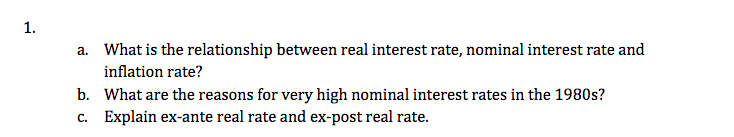a. What is the relationship between real interest rate, nominal interest rate and inflation rate? b. What are the reasons for very high nominal interest rates in the 1980s? c. Explain ex-ante real rate and ex-post real rate.

• ### A person received 4% nominal interest. The inflation rate was -2% and the tax rate was...

A person received 4% nominal interest. The inflation rate was -2% and the tax rate was 25%. This person received an after-tax real interest rate of 5%. Group of answer choices True False

• ### What is the real interest rate if the nominal interest rate is 8% and the expected...

What is the real interest rate if the nominal interest rate is 8% and the expected inflation rate is10% over the course of a year?